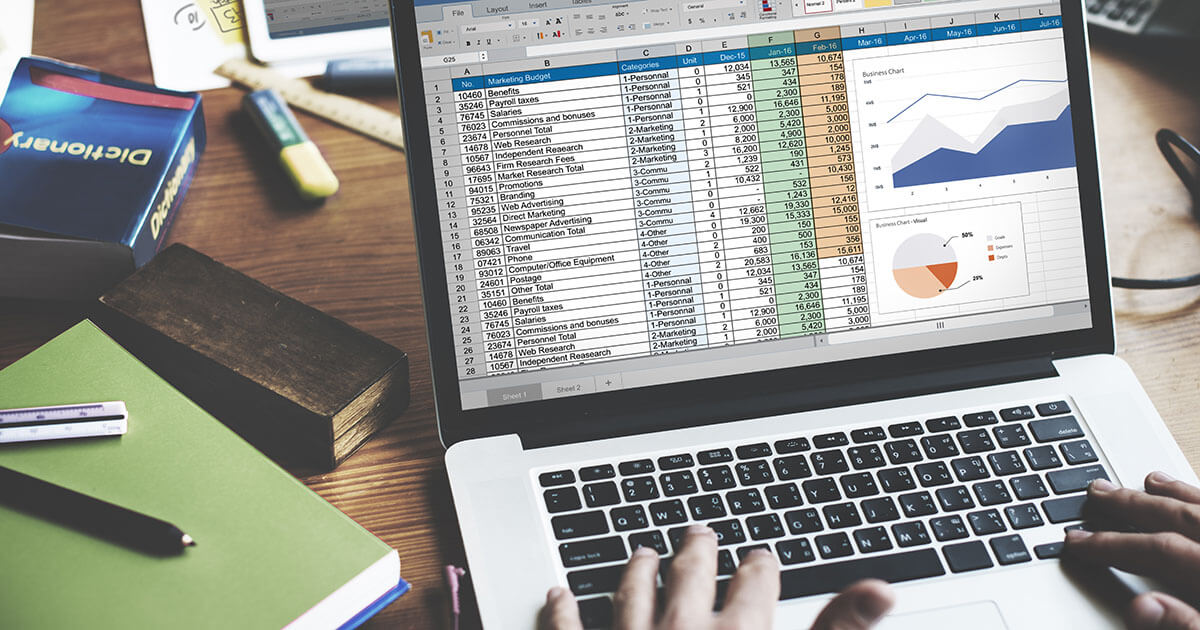# ExcelHow to use the Excel COUNT function
• Online sales

Analyses and calculations in Excel can be highly complex. Excel’s worksheets let you create huge tables, but sometimes you just want to answer a very simple question: How many cells in the table contain a number? In big worksheets, it can be virtually impossible to check the whole thing manually. The COUNT function in Excel was created specifically to solve this problem.

How to use the Excel COUNT functionHow to use the IF OR function in Excel
• Online sales

Using a combination of the IF function and the OR function, you can perform specific evaluations in Excel in which you can define multiple conditions in the same function. Therefore, the return value will be “TRUE” if either one of the conditions is met. We will explain when and how to use the IF OR formula in Excel using simple examples.

How to use the IF OR function in ExcelExcel OR function
• Online sales

Excel is an integral part of everyday business activities. Whether it is being used for tables, statistics or spreadsheets, this software simplifies complicated calculations and data analysis. The Excel OR function is one of the program’s easy-to-use commands. In this guide, we will explain and provide practical examples to illustrate how it can be used to make simple comparisons and check...

Excel OR functionExcel AND function: step-by-step instructions and examples
• Online sales

Excel offers over 400 useful functions. The AND function is one of the most popular ones. It allows you to check whether spreadsheet values meet certain conditions in just a few clicks. This article provides step-by-step instructions on how to use Excel AND with the help of an example. We will also show you how to get the most out of Excel by combining it with the IF function.

Excel AND function: step-by-step instructions and examplesThe Excel HLOOKUP function explained in simple terms
• Online sales

The HLOOKUP function in Excel is a useful feature for anyone who wants to look for specific values in a table. This requires all the compared values to be located in the same row (i.e. horizontally arranged). You can save a lot of time by using the HLOOKUP function, especially when dealing with large data sets, as our HLOOKUP example will illustrate.

The Excel HLOOKUP function explained in simple termsExcel: The “not equal to” operator explained
• Online sales

Excel is the perfect tool for evaluating large sets of data in tables and precisely defining conditions. Excel’s logical operators are particularly useful in this connection. In this article, you’ll learn how the Excel “not equal to” sign works and how it can be used with functions like IF.

Excel: The “not equal to” operator explainedEnabling Excel macros: How to activate these practical scripts
• Online sales

For over three decades, Microsoft has offered Excel, an excellent solution for creating simple spreadsheets and calculating complex formulas and functions. In addition to its vast functionality, another benefit of Excel spreadsheets is that you can create and use macros to automate any process or task. We’ll show you how to enable macros in Excel.

Enabling Excel macros: How to activate these practical scriptsSplitting Cells in Excel: How to Divide Cell Content Among Multiple Columns
• Online sales

If a table field contains several values, it often makes sense to split the respective Excel cell. Although the Microsoft spreadsheet program doesn’t offer a general function for this, you can split the cell content among multiple columns by configuring and using separators. We explain how you can use this option in your Excel document.

Splitting Cells in Excel: How to Divide Cell Content Among Multiple ColumnsSetting the print area in Excel
• Online sales

Excel spreadsheets can sometimes grow to epic proportions. However, you might not need the entire spreadsheet for a presentation. Rather than wasting tons of paper, you can set the print area in Excel. That way you can print out only the content that’s important to you right now. We’ll explain how to set and restore the print area in Excel.

Setting the print area in ExcelExcel: ROUNDDOWN – an explanation of this handy function
• Online sales

When performing complex calculations in tables, you can quickly produce values that are not fit for everyday use because they have far too many decimal places. The ROUNDDOWN function in Excel can help with this. It uses a simple formula to simplify your workflow. You can use it to round down any number to the desired number of decimal places.

Excel: ROUNDDOWN – an explanation of this handy function